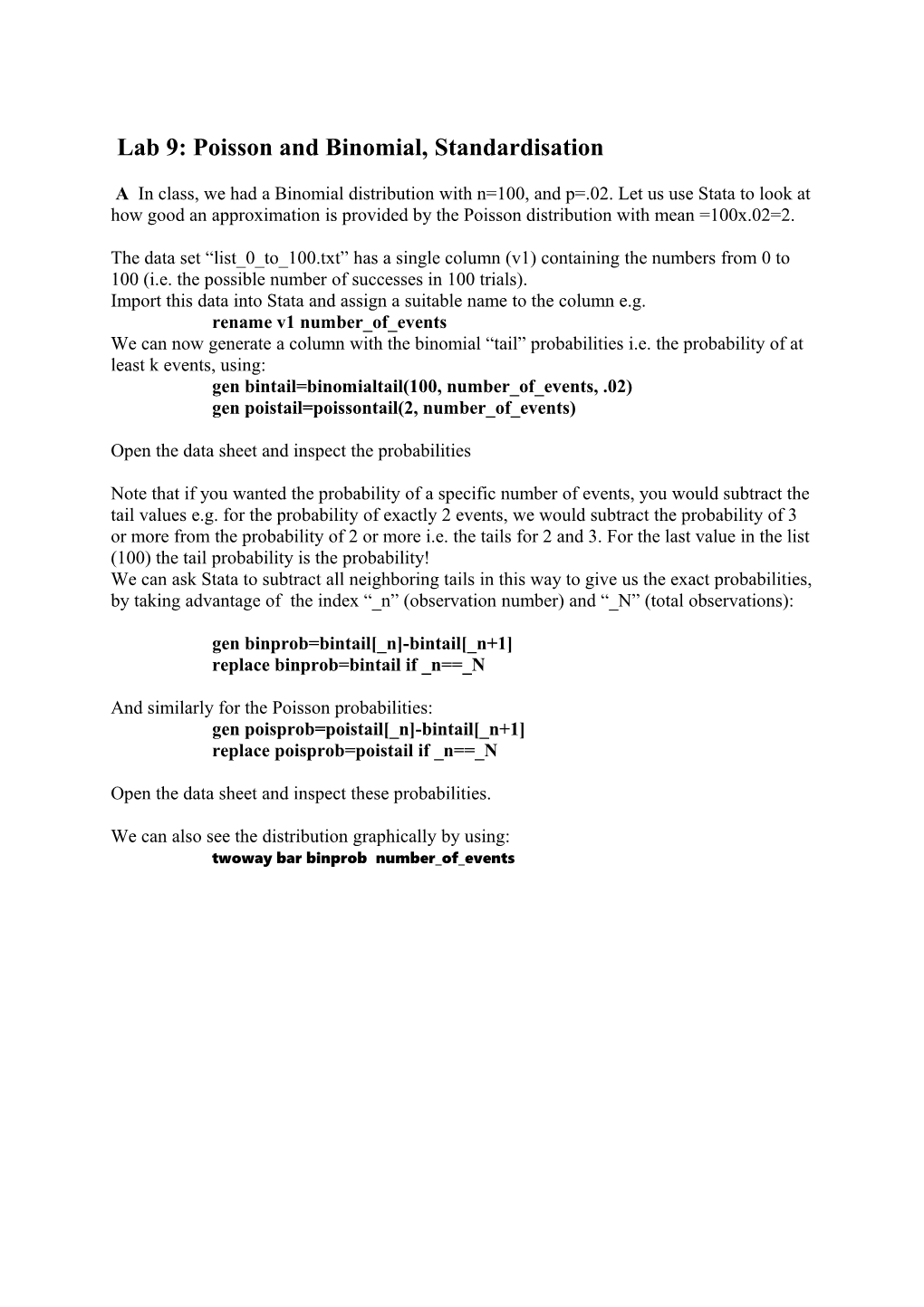# Lab 6: Stratification and StandardisationLab 9: Poisson and Binomial, Standardisation

A In class, we had a Binomial distribution with n=100, and p=.02. Let us use Stata to look at how good an approximation is provided by the Poisson distribution with mean =100x.02=2.

The data set “list_0_to_100.txt” has a single column (v1) containing the numbers from 0 to 100 (i.e. the possible number of successes in 100 trials).

Import this data into Stata and assign a suitable name to the column e.g.

rename v1 number_of_events

We can now generate a column with the binomial “tail” probabilities i.e. the probability of at least k events, using:

gen bintail=binomialtail(100, number_of_events, .02)

gen poistail=poissontail(2, number_of_events)

Open the data sheet and inspect the probabilities

Note that if you wanted the probability of a specific number of events, you would subtract the tail values e.g. for the probability of exactly 2 events, we would subtract the probability of 3 or more from the probability of 2 or more i.e. the tails for 2 and 3. For the last value in the list (100) the tail probability is the probability!

We can ask Stata to subtract all neighboring tails in this way to give us the exact probabilities, by taking advantage of the index “_n” (observation number) and “_N” (total observations):

gen binprob=bintail[_n]-bintail[_n+1]

replace binprob=bintail if _n==_N

And similarly for the Poisson probabilities:

gen poisprob=poistail[_n]-bintail[_n+1]

replace poisprob=poistail if _n==_N

Open the data sheet and inspect these probabilities.

We can also see the distribution graphically by using:

twoway bar binprob number_of_events

or since there is an almost zero probability for more than 10 events:

twoway bar binprob number_of_events if number_of_events <=10

and similarly for the Poisson distribution

or examine the probabilities on a scatter plot:

twoway scatter binprob poisprob

B. Open the FRACTURE.DTA dataset, which comes from a study of whether an inflatable device can prevent hip fractures among elderly people. Generate a variable for follow-up time (gen futime= time1-time0).

We can find the number of fractures and the total person time:

tabstat fracture futime, stat(sum)

stats | fracture futime

------+------

sum | 31 714

------

and we see that the incidence is 31/714

We can ask for a confidence interval for this rate, using Poisson:

ci fracture, exposure(futime) poisson

-- Poisson Exact --

Variable | Exposure Mean Std. Err. [95% Conf. Interval]

------+------

fracture | 714 .0434174 .007798 .0295 .0616275

Our main interest is theincidence rate ratio in those who use the protector versus those who do not, using the ir command

.

ir fracture protect futime

| wears device |

| Exposed Unexposed | Total

------+------+------

fracture event | 12 19 | 31

futime | 544 170 | 714

------+------+------

| |

Incidence rate | .0220588 .1117647 | .0434174

| |

| Point estimate | [95% Conf. Interval]

|------+------

Inc. rate diff. | -.0897059 | -.1414871 -.0379247

Inc. rate ratio | .1973684 | .0873718 .4282502 (exact)

Prev. frac. ex. | .8026316 | .5717498 .9126282 (exact)

Prev. frac. pop | .6115288 |

+------

(midp) Pr(k<=12) = 0.0000 (exact)

(midp) 2*Pr(k<=12) = 0.0000 (exact)

a)What are the values of the point estimates of the fracture incidence rate for the two groups (with and without the device)?

With: 0.022 cases per person-time;

Without: 0.111 cases per person-time.

b)What is the point estimate of the IRR? How is it interpreted?

IRR= 0.20. The risk was approximately 80% lower for people wearing devices compared to those not.

c)What is the incidence rate difference?

IRD= -0.09 with 95% CI -0.14 to -0.03.

d)What conclusions can you draw regarding the inflatable device?

Answer: The device is decreasing the incidence, The device is working good.

e)Calculate the IRR separately for patients of age 62-72 years of age and those 73-82 years of age. Do you think there is confounding from age? Do you think age is an effect modifier?

IRR 62-72: Inc. rate ratio | .1549296 | .0427589 .4724388 (exact)

IRR 73-82: Inc. rate ratio | .2010582 | .0601787 .6717397 (exact)

No confounding, no effect modification

C. Direct and Indirect Standardization

Direct standardization: rates of high blood pressure by city and year, using as standard the age, race, and sex distribution of all cities and years combined.

webuse hbp

generate pop = 1

dstdize hbp pop age race sex, by(city year)

Note that Stata provides detailed output, followed by a summary. Examine the data set and the output to understand what Stata is doing.

Indirect standardization: Obtain standardized mortality rates by state using the standard population saved in another data set (popkahn.dta)

webuse kahn, clear

istdize death pop age using by(state) pop(deaths pop)

Examine both data sets and the output to understand what Stata is doing